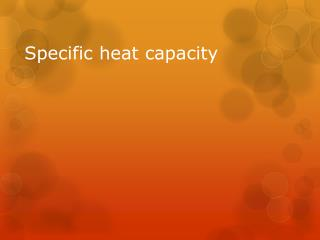DownloadDownload PresentationSpecific heat capacity

# Specific heat capacity

Télécharger la présentation## Specific heat capacity

- - - - - - - - - - - - - - - - - - - - - - - - - - - E N D - - - - - - - - - - - - - - - - - - - - - - - - - - -
##### Presentation Transcript

1. Specific heat capacity

2. Consider 2 beaker’s filled with paraffin and water 25c 50C 75C 25c Hot plate paraffin Water If both are heated on the same heat source, over the same amount of time, the temperature rise of the paraffin will be twice as high.

3. What is specific heat capacity? • The paraffin heats up twice as much given the same amount of energy as the water does. We say that the specific heat capacity or the amount of energy it takes to raise the temperature of the water is higher than the specific heat capacity of the paraffin. • Definition: “specific heat capacity of a substance is the heat used to produce unit temperature rise given unit mass” • Specific heat capacity is usually quoted as the energy(j) absorbed to raise the Temperature of a mass of 1 Kg by 1 K or 1 Degree Celsius. It’s units are J Kg-1 K-1 or c-1 and it’s symbol is (c).

4. What is specific heat capacity? E.g If a substance has a specific heat capacity of 1000J Kg-1C-1 Then applying 1000J of heat energy will cause 1 kg of that substance to increase it’s temperature by 1 degree. Applying 2000J increases the temperature by 2C. It could also be said that applying 1000J will cause the temperature of 0.5 kg of that substance to rise 2C.

5. Please note that the specific heat capacity is also the amount of heat energy which a kilogram of that substance will release to the environment for every degree drop in temperature. • E.g A substance with a specific heat capacity of 1000Jkg-1C-1 will release 1000J if the temperature of 1Kg of the substance decreases from 25C to 24C and 10,000J if it’s temperature decreases from 24C to 14C.

6. The Heat equation • Heat received or given out = mass x temp. change x specific heat capacity. • Eheat= m x Δθ x c • EH= heat energy • Δ= Change of • Θ= temperature • c= specific heat capacity

7. Practice: • How much heat is given out when an iron ball of mass 2 Kg and specific heat capacity 440 Jkg-1C-1 cools from 300 to 200C? 88,000J

8. A piece of aluminium of mass 0.5Kg is heated to 100C and then placed in 0.4kg of water at 10C. If the resulting temperature of the mixture is 30C, what is the specific heat capacity of aluminium if that of water is 4,200Jkg-1c-1? 960Jkg-1C-1